Get instant live expert help with Excel or Google Sheets“My Excelchat expert helped me in less than 20 minutes, saving me what would have been 5 hours of work!”

#### Post your problem and you'll get expert help in seconds

Your message must be at least 40 characters
Our professional experts are available now. Your privacy is guaranteed.

# Excel POWER Function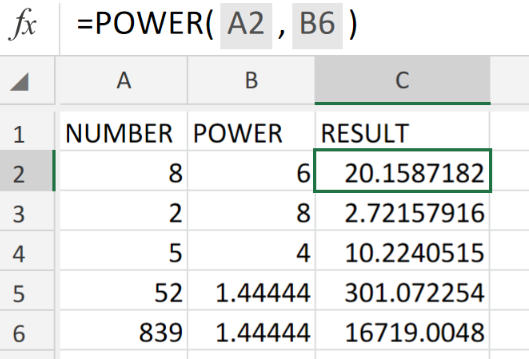Figure 1. of Excel POWER Function.

In the event that we want to determine a very small level of tolerance for vast distances. At some point we will have to raise a number to a specific power. We are going to utilize the Excel POWER Function. This article will walk through how to do it.

## Generic Formula

`POWER(number, power)`

The POWER Function will calculate a number raised to a specific power. The formula syntax has the following components;

• number – the number value which we desire to raise to a certain power. This can be any real number.
• power – the exponential value to which we are going to raise our base number.

## How to use the Excel POWER Function.

We can achieve this by following three simple steps!

1. Collect the data available to us for checking, in our Excel sheet. See example illustrated below;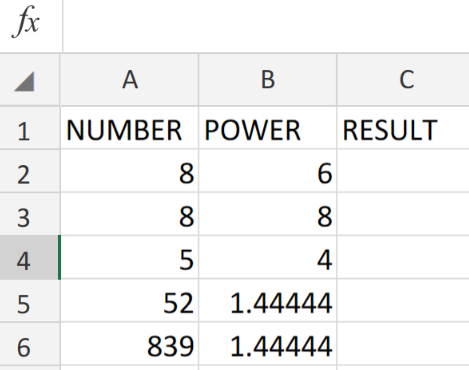Figure 2. of Number Values for the POWER Function in Excel.

1. Our purpose here is to exponentially raise the number values in column A to the power Values specified in column B of our worksheet. The formula syntax which we will enter into the formula bar for cell C2, for the desired result is as follows;

`=POWER(A2,B6)`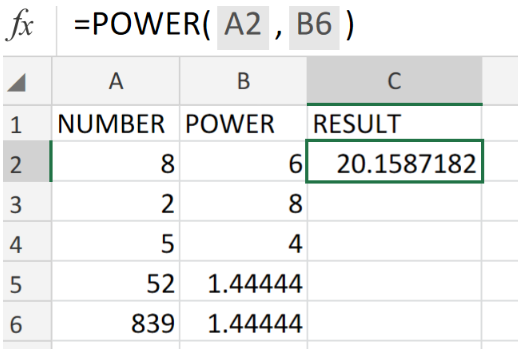Figure 3. of the POWER Function in Excel.

1. Modify and copy the formula syntax into the other cells in column C of the example illustrated below to achieve the desired results.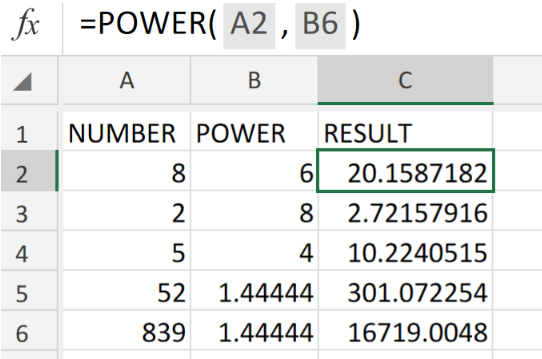Figure 4. of the POWER Function in Excel.

The POWER Function operates like an exponential value in a basic mathematical equation.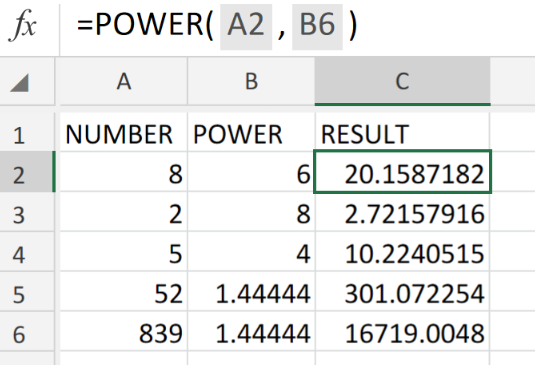Figure 5. of Final Result.

## Instant Connection to an Expert through our Excelchat Service

Our live Excelchat Service is here for you. We have Excel Experts available 24/7 to answer any Excel questions you may have. Guaranteed connection within 30 seconds and a customized solution for you within 20 minutes.

### Did this post not answer your question? Get a solution from connecting with the expert.Another blog reader asked this question today on Excelchat:## Subscribe to Excelchat.coAnother blog reader asked this question today on Excelchat: